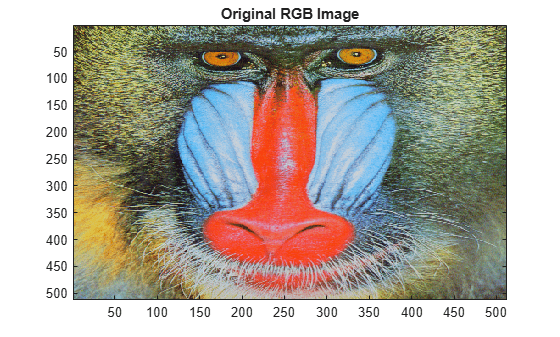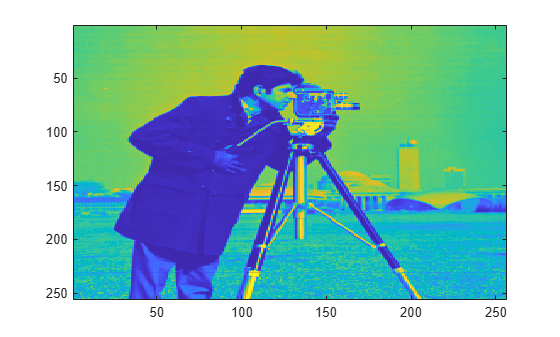Main Content

# ihaart2

Inverse 2-D Haar wavelet transform

## Syntax

``xrec = ihaart2(a,h,v,d)``
``xrec = ihaart2(a,h,v,d,level)``
``xrec = ihaart2(___,integerflag)``

## Description

example

````xrec = ihaart2(a,h,v,d)` returns the inverse 2-D Haar transform, `xrec`, for the approximation coefficients, `a`, and the horizontal, vertical, and diagonal detail coefficients, `h`, `v`, and `d`. All the inputs, `a`, `h`, `v`, and `d`, are outputs of `haart2`. ```

example

````xrec = ihaart2(a,h,v,d,level)` returns the inverse 2-D Haar transform at the specified level. ```

example

````xrec = ihaart2(___,integerflag)` specifies how the inverse 2-D Haar transform handles integer-valued data, using any of the previous syntaxes.```

## Examples

collapse all

Obtain the inverse 2-D Haar transform of image and view the reconstructed image.

Load the image and obtain its 2-D Haar transform.

```im = imread('mandrill.png'); [a,h,v,d] = haart2(im);```

Use the inverse 2-D Haar transform to reconstruct the image.

`xrec = ihaart2(a,h,v,d);`

Compare the original and reconstructed images.

```imagesc(im) title('Original RGB Image')``````figure imagesc(uint8(xrec)) title('Reconstructed RGB Image')```Obtain the 2-D Haar transform of an image limiting the transform to 2 levels.

Load and view the image of a cameraman.

```im = imread('cameraman.tif'); imagesc(im)```Obtain the 2-D Haar transform using the default maximum number of levels.

`[a,h,v,d] = haart2(im);`

Reconstruct the image using the inverse 2-D Haar transform and view the image. Notice the near-perfect reconstruction.

```xrec = ihaart2(a,h,v,d); imagesc(xrec)```Reconstruct and view the image using the inverse 2-D Haar transform, limited to level 2. Level 2 corresponds to the fourth scale because scale is defined as ${2}^{j}$, where j is the level.

```xrec1 = ihaart2(a,h,v,d,2); imagesc(xrec1)```Using fewer levels returns the average of the original image at level 2.

Obtain the 2-D Haar transform of an image limiting the transform to integer data.

Load the image of a cameraman.

`im = imread('cameraman.tif');`

Obtain the 2-D Haar transform using the `'integer'` flag.

`[a,h,v,d]=haart2(im,'integer');`

Reconstruct the image using the inverse 2-D Haar transform and view the image.

```xrec = ihaart2(a,h,v,d,'integer'); imagesc(xrec)```Use integer data when you need to reduce the amount of memory used compared to noninteger data.

## Input Arguments

collapse all

Approximation coefficients, specified as a scalar or matrix of coefficients, depending on the level to which the 2-D Haar transform was calculated. `a` is an output from the `haart2` function. Approximation, or scaling, coefficients are a lowpass representation of the input. If `a` and the elements of `h`, `v`, and `d`, are vectors, `xrec` is a vector. If `a` and the elements of `h`, `v`, and `d` are matrices, `xrec` is a matrix, where each column is the inverse 2-D Haar transform of the corresponding columns in `a` and `h`, `v`, or `d`.

Data Types: `single` | `double`

Horizontal detail coefficients by level, specified as a matrix or cell array of matrices. `h` is an output from the `haart2` function. If `h` is a matrix, the 2-D Haar transform was computed only down to one level coarser in resolution.

Data Types: `single` | `double`

Vertical detail coefficients by level, specified as a matrix or cell array of matrices. `v` is an output from the `haart2` function. If `v` is a matrix, the 2-D Haar transform was computed only down to one level coarser in resolution.

Data Types: `single` | `double`

Diagonal detail coefficients by level, specified as a matrix or cell array of matrices. `d` is an output from the `haart2` function. If `d` is a matrix, the 2-D Haar transform was computed only down to one level coarser in resolution.

Data Types: `single` | `double`

Maximum level to which to invert the Haar transform, specified as a nonnegative integer. If `h` is a cell array, `level` is less than or equal to `length(h)-1`. If `h` is a vector or matrix, `level` must equal `0` or be unspecified.

Integer-valued data handling, specified as either `'noninteger'` or `'integer'`. `'noninteger'` does not preserve integer-valued data in the 2-D Haar transform, and `'integer'` preserves it. The `'integer'` option applies only if all elements of inputs, `a`, `h`, `v`, and `d`, are integer-valued. The inverse 2-D Haar transform algorithm, however, uses floating-point arithmetic.

## Output Arguments

collapse all

2-D Haar wavelet transform, returned as a matrix.

Data Types: `single` | `double`

## See Also

Introduced in R2016b

## SupportGet trial now